# 3. Two axis aligned very long,thin shell cylinders have radii Ri and R2 with Ri 〈...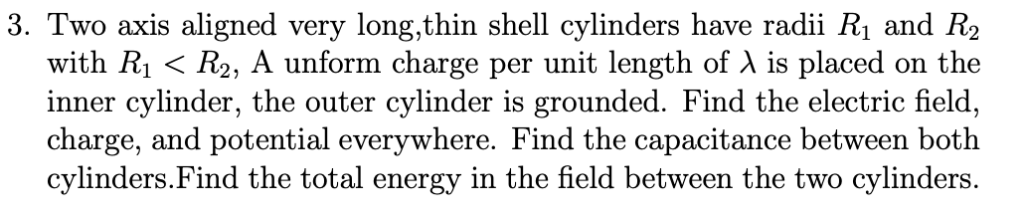3. Two axis aligned very long,thin shell cylinders have radii Ri and R2 with Ri 〈 R2, A unform charge per unit length of λ is placed on the inner cylinder, the outer cylinder is grounded. Find the electric field, charge, and potential everywhere. Find the capacitance between both cylinders.Find the total energy in the field between the two cylinders.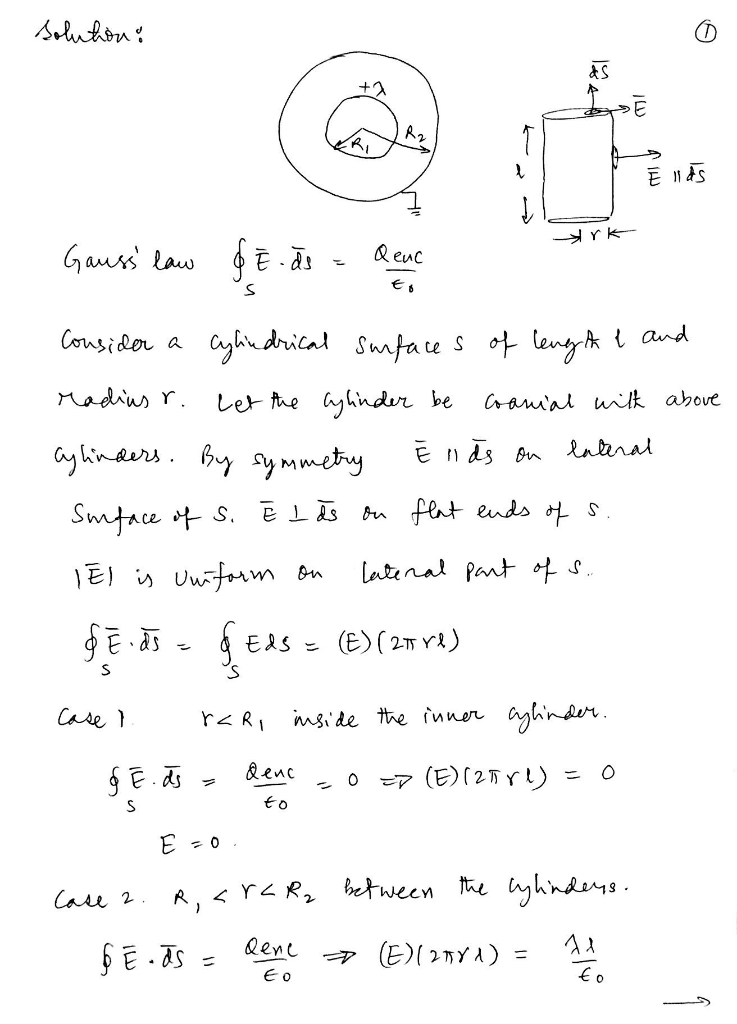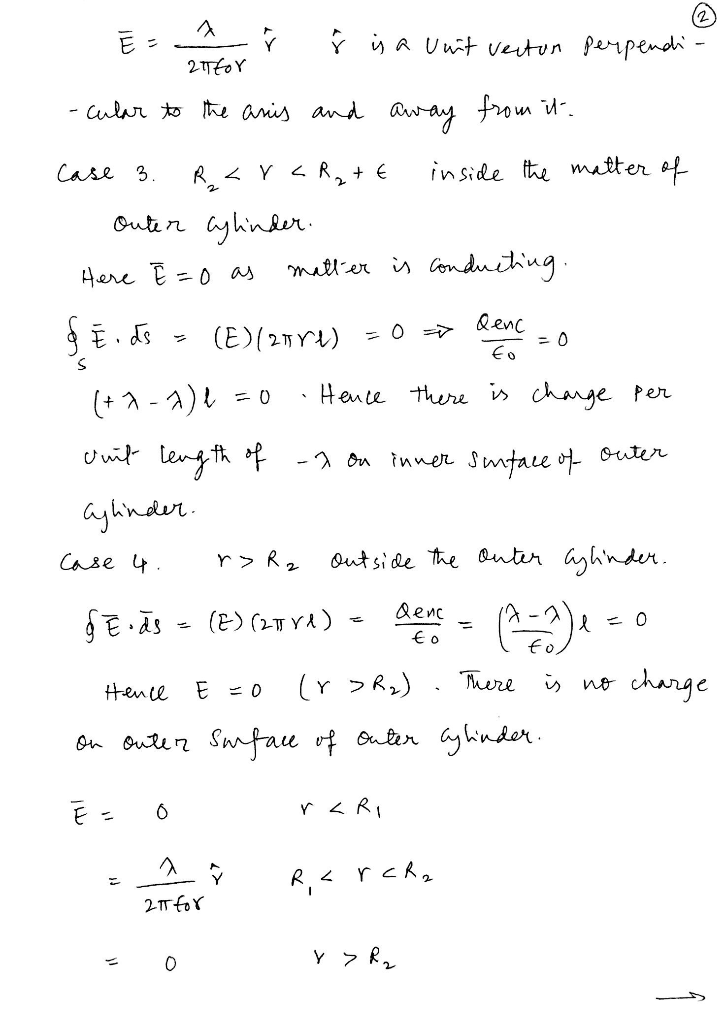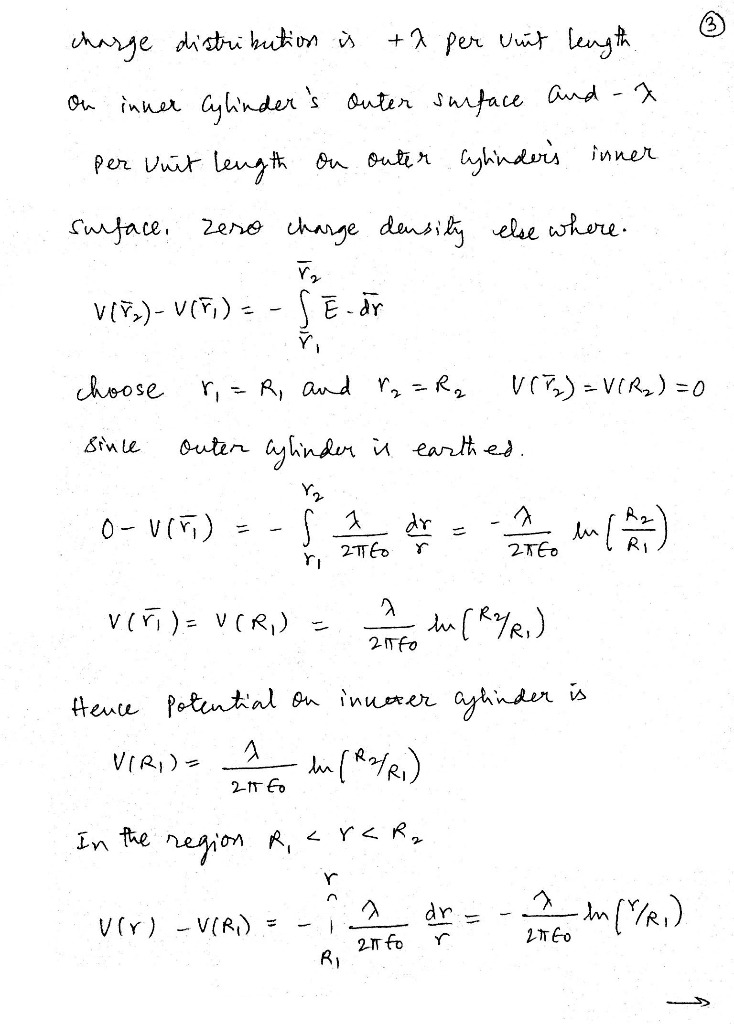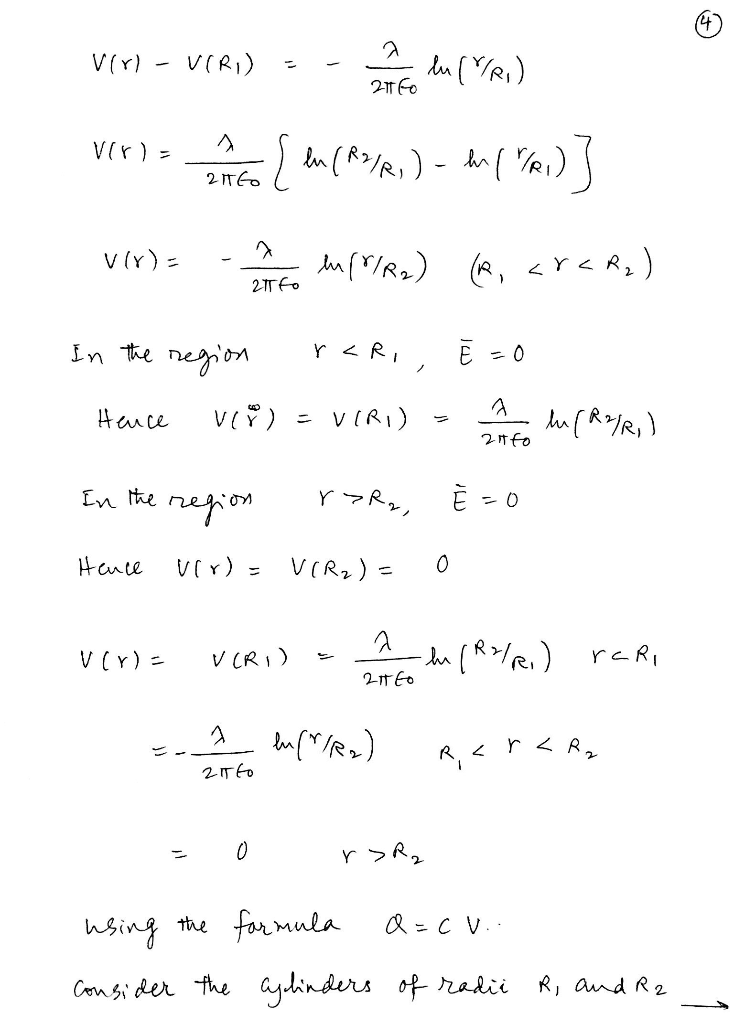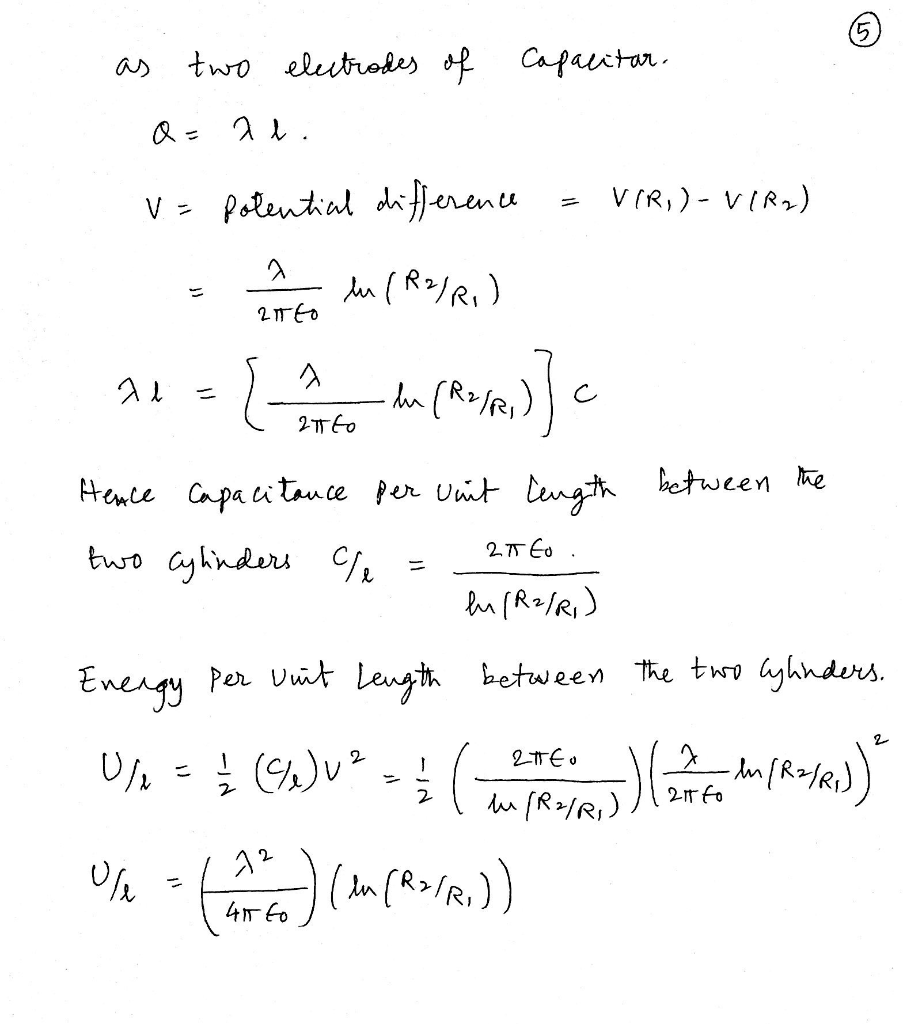#### Earn Coin

Coins can be redeemed for fabulous gifts.

Similar Homework Help Questions
• ### Two very long concentric conducting cylindrical shells are arranged as seen below. The inner shell has...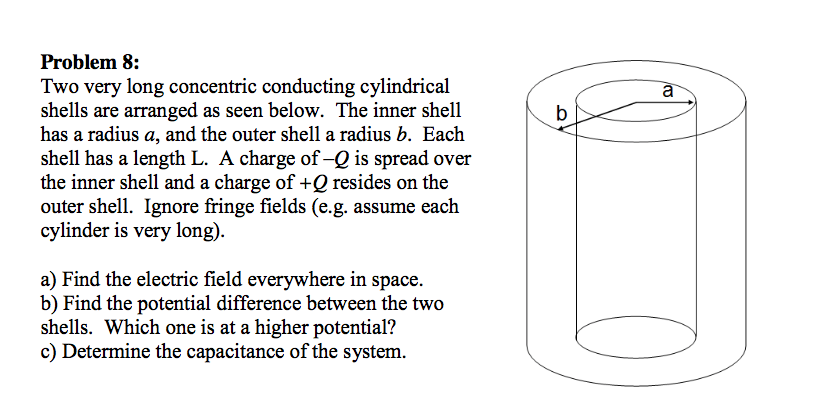Two very long concentric conducting cylindrical shells are arranged as seen below. The inner shell has a radius a, and the outer shell a radius b. Each shell has a length L. A charge of -Q is spread over the inner shell and a charge of +Q resides on the outer shell. Ignore fringe fields (e.g. assume each cylinder is very long). Find the electric field everywhere in space. Find the potential difference between the two shells. Which one is...

• ### Two long, charged, thin-walled, concentric cylinders have radii of 3.0 and 6.0 cm. The charge per...

Two long, charged, thin-walled, concentric cylinders have radii of 3.0 and 6.0 cm. The charge per unit length is 4.9 ✕ 10- 6 C/m on the inner shell and -8.5 ✕ 10-6 C/m on the outer shell. (a) Find the magnitude and direction of the electric field at radial distance r = 4.9 cm from the common central axis. (Take radially outward to be positive.) __________ N/C (b) Find the magnitude and direction of the electric field at r =...

• ### P's The diagram above shows a cross-section view of a very long straight metal cylinder of radius...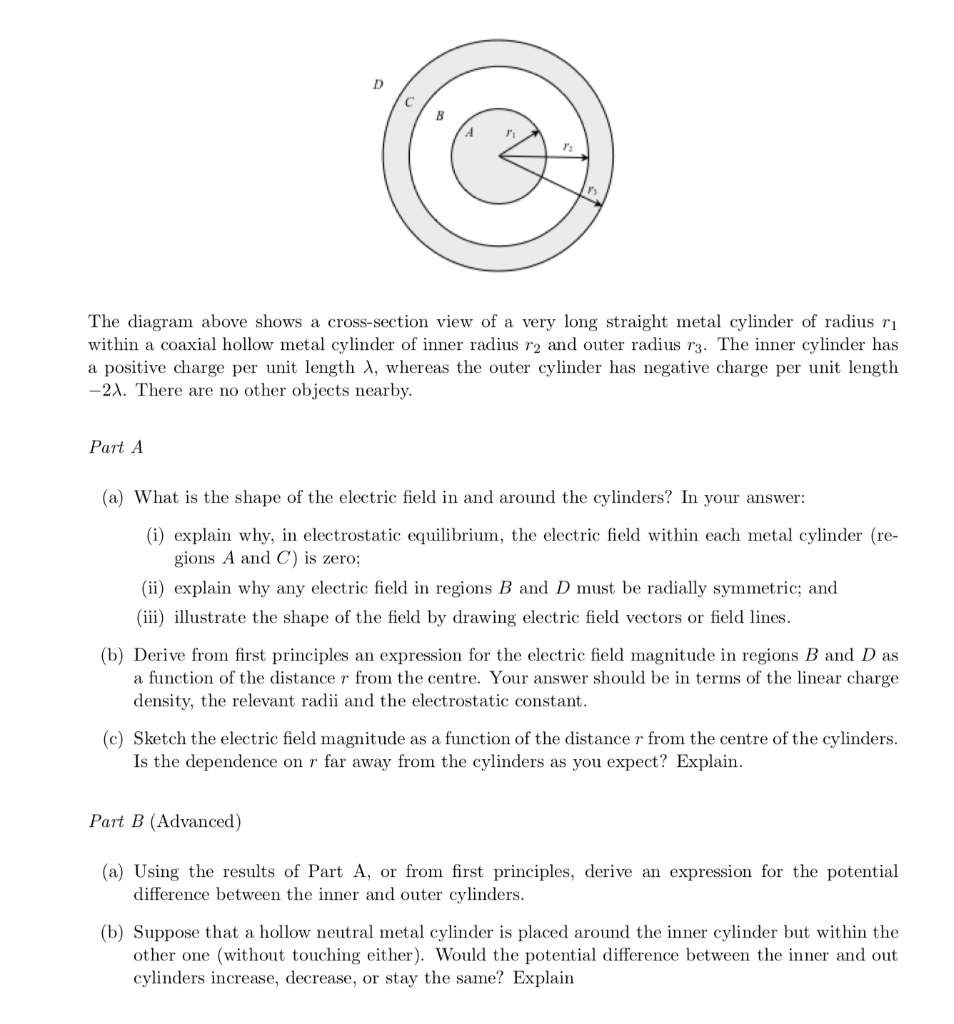P's The diagram above shows a cross-section view of a very long straight metal cylinder of radius ri within a coaxial hollow metal cylinder of inner radius r2 and outer radius r3. The inner cylinder has a positive charge per unit length λ, whereas the outer cylinder has negative charge per unit length There are no other objects nearby Part A (a) What is the shape of the electric field in and around the cylinders? In your answer (i) explain...

• ### 2. (25 points) Two concentric spheres have radii Ri and R2 (R2 > Ri). The potential...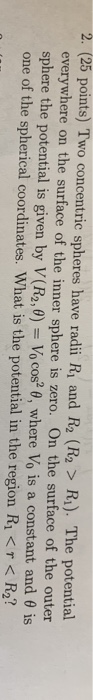2. (25 points) Two concentric spheres have radii Ri and R2 (R2 > Ri). The potential everywhere on the surface of the inner sphere is zero. On the surface of the outer sphere the potential is given by V(R2,0-10 cos2 θ, where Vo is a constant and θ is one of the spherical coordinates. What is the potential in the region Ri < R2?

• ### Consider two thin, concentric conducting spherical shells with radii r1 = 0.50 m and r2 =...

Consider two thin, concentric conducting spherical shells with radii r1 = 0.50 m and r2 = 1.0 m. A charge of +1.0 mC is placed on the inner sphere and a charge of +2.0 mC is placed on the outer sphere. Set the potential at infinity to be 0. Determine (a) the field inside the inner sphere, (b) the charge on the inner surface of the outer conductor, (c) the magnitude of the E-field midway between the inner and outer...

• ### A variable capacitor consists of two thin coaxial metal cylinders of radii a and b, with...

A variable capacitor consists of two thin coaxial metal cylinders of radii a and b, with (b - a) << a, free to move with respect to each other in the axial direction. The length of the cylinders is L, and the potential difference between the two cylinders is V. Initially, the inner cylinder (radius = a) is completely enclosed by the outer cylinder (radius = b). Using energy methods, find the magnitude and direction of the force on the...

• ### The diagram above shows a cross-section view of a very long straight metal cylinder of radius ri ...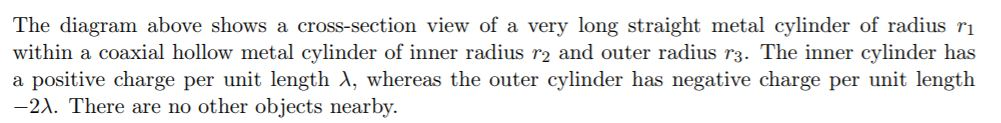The diagram above shows a cross-section view of a very long straight metal cylinder of radius ri within a coaxial hollow metal cylinder of inner radius r2 and outer radius r3. The inner cylinder has a positive charge per unit length λ, whereas the outer cylinder has negative charge per unit length 2A. There are no other objects nearby. Derive from first principles an expression for the electric field magnitude in regions B and D as a function of the...

• ### 4. The accompanying ve shows two concentric spherical shells of negligible thickness and Radii Ri and...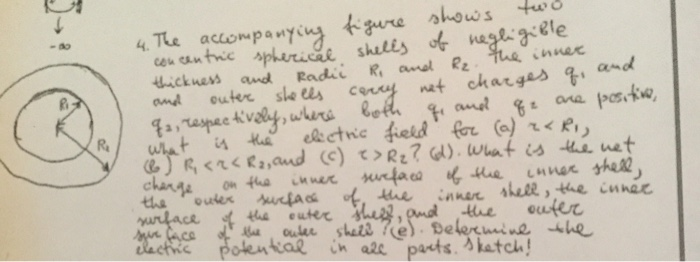4. The accompanying ve shows two concentric spherical shells of negligible thickness and Radii Ri and R₂. the inner and outer shells carry not charges q, and qa, respectively, where both qe and qe are positive, What is the electric field for (a) rari, 6 RC2CR2, and (c) ?> R2? (d). What is the net charge on the inner surface of the inner shell, the outer surface of the inner shell, the surface inner of the outer shes, and the...

• ### Please Justify Answers and Show All work. 3. Two concentric spherical metal shells have radii a...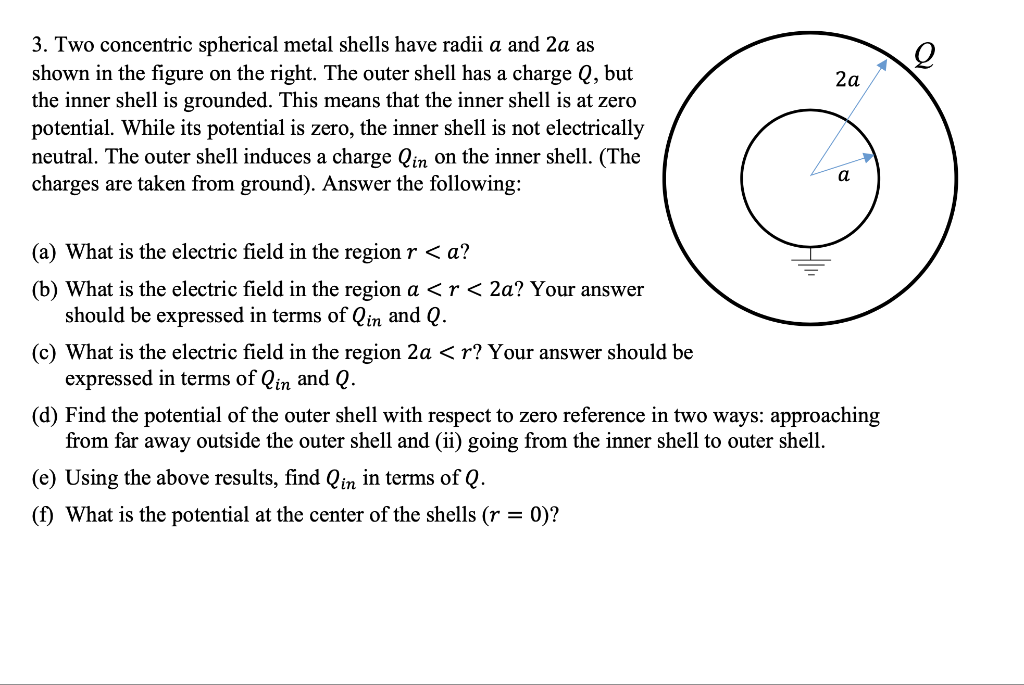Please Justify Answers and Show All work. 3. Two concentric spherical metal shells have radii a and 2a as shown in the figure on the right. The outer shell has a charge Q, but the inner shell is grounded. This means that the inner shell is at zero potential. While its potential is zero, the inner shell is not electrically neutral. The outer shell induces a charge lin on the inner shell. (The charges are taken from ground). Answer the...

• ### 10ASond cylinder with a charge per unit length of 21 and a radius of Ri is surrounded by a thin c...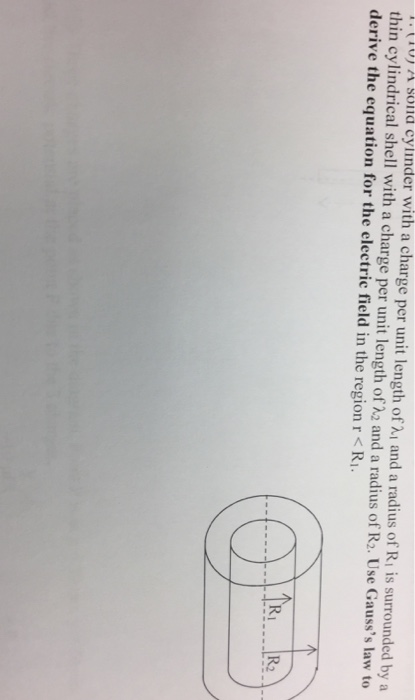10ASond cylinder with a charge per unit length of 21 and a radius of Ri is surrounded by a thin cylindrical shell with a charge per unit length of λ 2 and a radius of R2. Use Gauss's law to derive the equation for the electric field in the region r